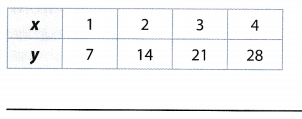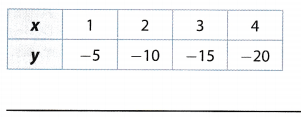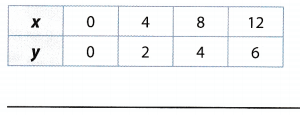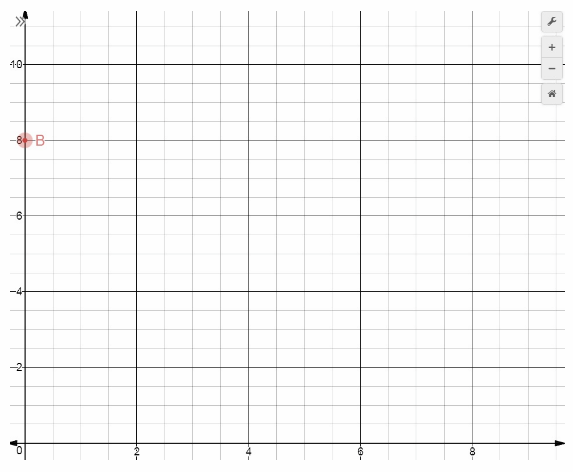# Texas Go Math Grade 6 Module 14 Answer Key Relationships in Two Variables

Refer to our Texas Go Math Grade 6 Answer Key Pdf to score good marks in the exams. Test yourself by practicing the problems from Texas Go Math Grade 6 Module 14 Answer Key Relationships in Two Variables.

## Texas Go Math Grade 6 Module 14 Answer Key Relationships in Two Variables

Essential Question
How can you use relationships in two variables to solve real-world problems?

Complete these exercises to review skills you will need for this chapter.

Multiply.

Question 1.
7 × 6 ____
Solution to this example is given below
7 × 6 =? Use a related fact you know.
6 × 6 = 36.
Think:7 × 6 = (6 × 6) + 6
= 36 + 6
= 42
Final solution
42

Question 2.
10 × 9 ____
Solution to this example is given below
10 × 9 = 7 Use a related fact you know.
9 × 9 = 81.
Think: 10 × 9 = (9 × 9) + 9
= 81 + 9
= 90
Final solution
90

Question 3.
13 × 12 ____
Solution to this example is given below
13 × 12 =? Use a related fact you know.
12 × 12 = 144.
Think: 13 × 12 = (12 × 12) + 12
= 144 + 12
= 156
Final solution
156

Question 4.
8 × 9 ____
Solution to this example is given below
8 × 9 =? Use a related fact you know.
8 × 8 = 64.
Think: 8 × 9 = (8 × 8) + 8
= 64 + 8
= 72
Final solution
72

Write the rule for each table.

Question 5.Compare the x- and y-values to find a pattern.
Each x-value is 7 times the corresponding y-value
Use the pattern to write an equation expressing y in terms of x
y = 7x
y = 7x Final solution
y = 7x

Question 6.Compare the x- and y-values to find a pattern.
Each x-value is 6 more than the corresponding y-value
Use the pattern to write an equation expressing y in terms of x
y = x + 6
y = x + 6 Final solution
y = x + 6

Question 7.For example let x1 be 1 and x2 be 3 and because of this y1 will be -5 and y2 will be -15. We will use equation of a line through those two points and get:
y – y1 = $$\frac{y_{2} \cdot y_{1}}{x_{2}-x_{1}}$$(x – x1)
y + 5 = $$\frac{-15+5}{3-1}$$(x – 1)
y + 5 = -5(x – 1)
y = -5x + 5 – 5
y = -5x
So,
required rute is y = -5x.
y = -5x

Question 8.Compare the x- and y-values to find a pattern.
Each x-value is 0.5 times the corresponding y-value
Use the pattern to write an equation expressing y in terms of x
y = 0.5x
y = 0.5x Final solution
y = 0.5x

Graph each point on the coordinate grid above.

Question 9.
B (0, 8)
Solution to this example is given below
Start at the origin.
Move 0 units right
Then move 8 units up.
Graph point B( 0, 8).Question 10.
C (2, 3)
Solution to this example is gin below
Start at the origin
Move 2 units right
Then move 3 units up.
Graph point C( 2, 3).Question 11.
D (6, 7)
Solution to this example is given below
Start at the origin.
Move 6 units right
Then move 7 units up.
Graph point D( 6, 7).Question 12.
E (5, 0)
Solution to this example is given below
Start at the origin.
Move 5 units right
Then move 0 units up.
Graph point E( 5, 0).Visualize Vocabulary
Use the ✓ words to complete the chart.Understand Vocabulary

Complete the sentences using the preview words.

Question 1.
The numbers in an ordered pair are _____